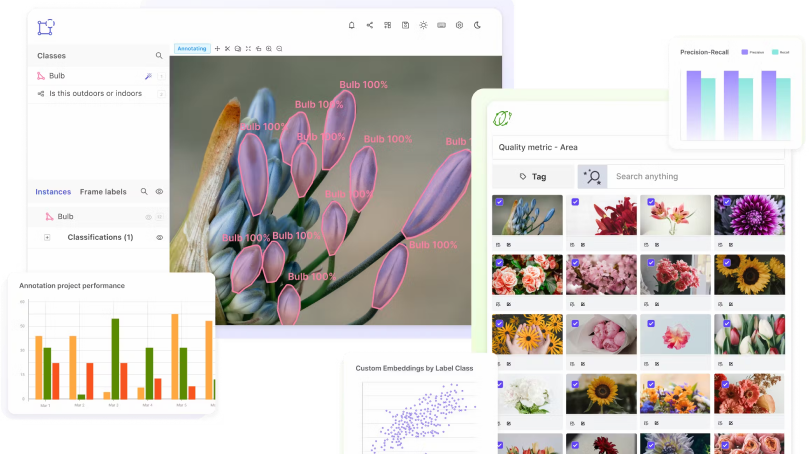# Normalization

Encord Computer Vision Glossary

## Normalization

Normalization is a process in which the values of a dataset are scaled and transformed to have a common scale, without distorting differences in the rank or order of the values. It is a common preprocessing step in machine learning (ML) and is used to ensure that the data is in a consistent format and is suitable for use with ML algorithms.

Normalization can be done in a variety of ways, including standardization, z-score normalization, and min-max normalization. By scaling the data, min-max normalization ensures that all of the values fall between a predetermined minimum and maximum value, typically 0 and 1. Data that has undergone standardization is scaled to have a mean of 0 and a standard deviation of 1. By applying the z-score formula, z-score normalization scales the data to have a mean of 0 and a standard deviation of 1.

Normalization is often used to ensure that the data is in a consistent format and is suitable for use with ML algorithms, as many algorithms assume that the data is normally distributed and that the features are on a similar scale. Normalization can also help to reduce the impact of outliers and can improve the performance of some algorithms.

Overall, normalization is an important preprocessing step in ML, and is used to ensure that the data is in a consistent format and is suitable for use with ML algorithms. It can help to improve the performance of the algorithms and can reduce the impact of outliers.

Scale your annotation workflows and power your model performance with data-driven insights## What are methods for doing normalization for computer vision?

Normalization can be done in a variety of ways, including standardization, z-score normalization, and min-max normalization. By scaling the data, min-max normalization ensures that all of the values fall between a predetermined minimum and maximum value, typically 0 and 1. Data that has undergone standardization is scaled to have a mean of 0 and a standard deviation of 1. By applying the z-score formula, z-score normalization scales the data to have a mean of 0 and a standard deviation of 1.

Normalization is often used to ensure that the data is in a consistent format and is suitable for use with ML algorithms, as many algorithms assume that the data is normally distributed and that the features are on a similar scale. Normalization can also help to reduce the impact of outliers and can improve the performance of some algorithms.

Overall, normalization is an important preprocessing step in ML, and is used to ensure that the data is in a consistent format and is suitable for use with ML algorithms. It can help to improve the performance of the algorithms and can reduce the impact of outliers.#### Discuss this blog on Slack

Join the Encord Developers community to discuss the latest in computer vision, machine learning, and data-centric AI

Join the community
###### Subscribe